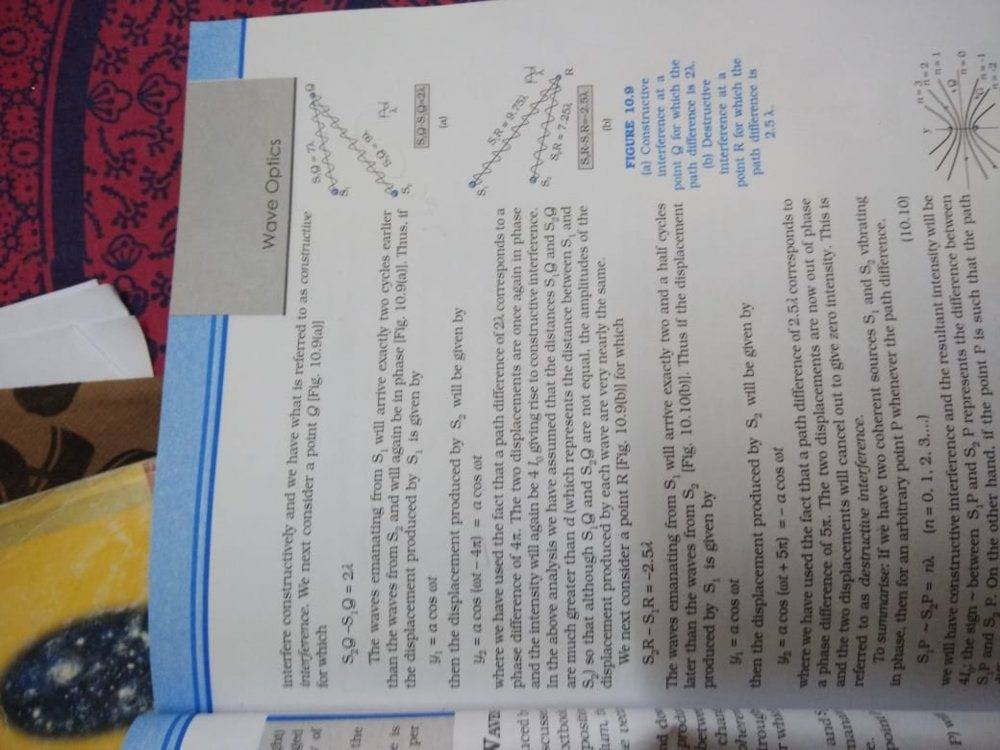# Waves phase difference

Crystal037
Homework Statement:
Here we have subtracted the phase 4pi from S2 than S1. Why
Relevant Equations:
y=a cos (wt)See fig(a), S1Q=7lambda
S2Q=9lambda
I think since source S2 is lagging behind. So, we should add the phase 4pi instead of subtracting it from the cosine function. Wouldn't subtracting the phase further delay the wave more.

Last edited:
•Delta2

## Answers and Replies

Gold Member
I vote for a very poorly worded explanation! Using the Fig 10.9(a) I agree with your statement.

Unfortunately, if you look at their describing equation (S2- S1=2λ, near the top of the page) the equations they give are consistent.

edit: Fortunately in this case it doesn't matter because the sign of the phase difference doesn't matter here. That's the usual case when working with optics. If working with electrical signals (for instance comparing two signals) it may make a difference. /edit:

Just another case of poor copy editing on the part of the book publisher.

Cheers,
Tom

p.s. Good catch Crystal!

Crystal037
So am I right that y2=a cos(wt+4pi)

Gold Member
So am I right that y2=a cos(wt+4pi)
Yes. See also the edit/insertion to my first response.

Cheers,
Tom

Crystal037
Thanks Tom. G but how will it make a difference in electrical signal.

Gold Member
One example would be in data transmission using Phase Shift Keying (PSK). This method uses a fixed frequency transmitting data but varies the phase dependent on the data value at that instant.

Notice that the phase is shifted by 180° as the data changes between '0' and '1'.

(I hope the image shows, it doesn't appear in my Preview. It's the big image on the right of the link with a Black background.)

image found with: